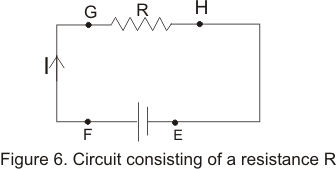# Electric energy|Electric power

## Electric Energy and Power

• To understand the process of energy transfer in a simple circuit consider a simple circuit as shown in the figure given below• Positive terminal of the battery as we all know is always at higher potential.
• Let ΔQ amount of charge begin to flow in the circuit from point E through the battery and resistor and then back to point E.
• When Charge ΔQ moves from point E to point F through the battery electric potential potential energy of the system increased by the amount
ΔU=ΔQ V -(6) and the electric energy of the battery decreased by the same amount.
• When charge ΔQ moves from point G to S through resistor R, there comes a decrease in electric potential energy.
• This loss in potential energy appears as the increased in thermal energy of the resistor.
• Thermal energy of the resistor increases because when the charge moves through the resistor they loss there electrical potential energy by colliding with the atom in the resistor. This way electrical energy is transformed internal energy corresponding to increase in vibrational motion of the atom of the resistor and this cause increase in temperature of the resistor.
• The connecting wires are assumed to have negligible resistance and no energy transfer occur for the path FG and HE.
• In time Δt charge ΔQ moves through the resistor i.e. from G to H. The rate at which it loss potential energy
ΔU/Δt=(ΔQ/Δt)ΔV=IΔV where I is the current in the resistor and ΔV is the potential difference across it.
• This charge ΔQ regain its energy when it passes through the battery at the cost of conservation of chemical energy of electrolyte to the electrical energy.
• This loss of potential energy as stated earlier appears as increased thermal energy of the resistor. If P represents the rate at which energy is delivered to the resistor then
P=IΔV
• We know that ΔV =IR for a resistor hence alternative forms of equation(8) are
P=I2R=ΔV2/R where I is expressed in amperes, ΔV iv volts and resistance R in ohm(Ω)
• SI unites of power is watt such that
1 watt=1 volt* 1 Ampere
Bigger unit of electric power are Kilowatt(KW) and Megawatt(MW).